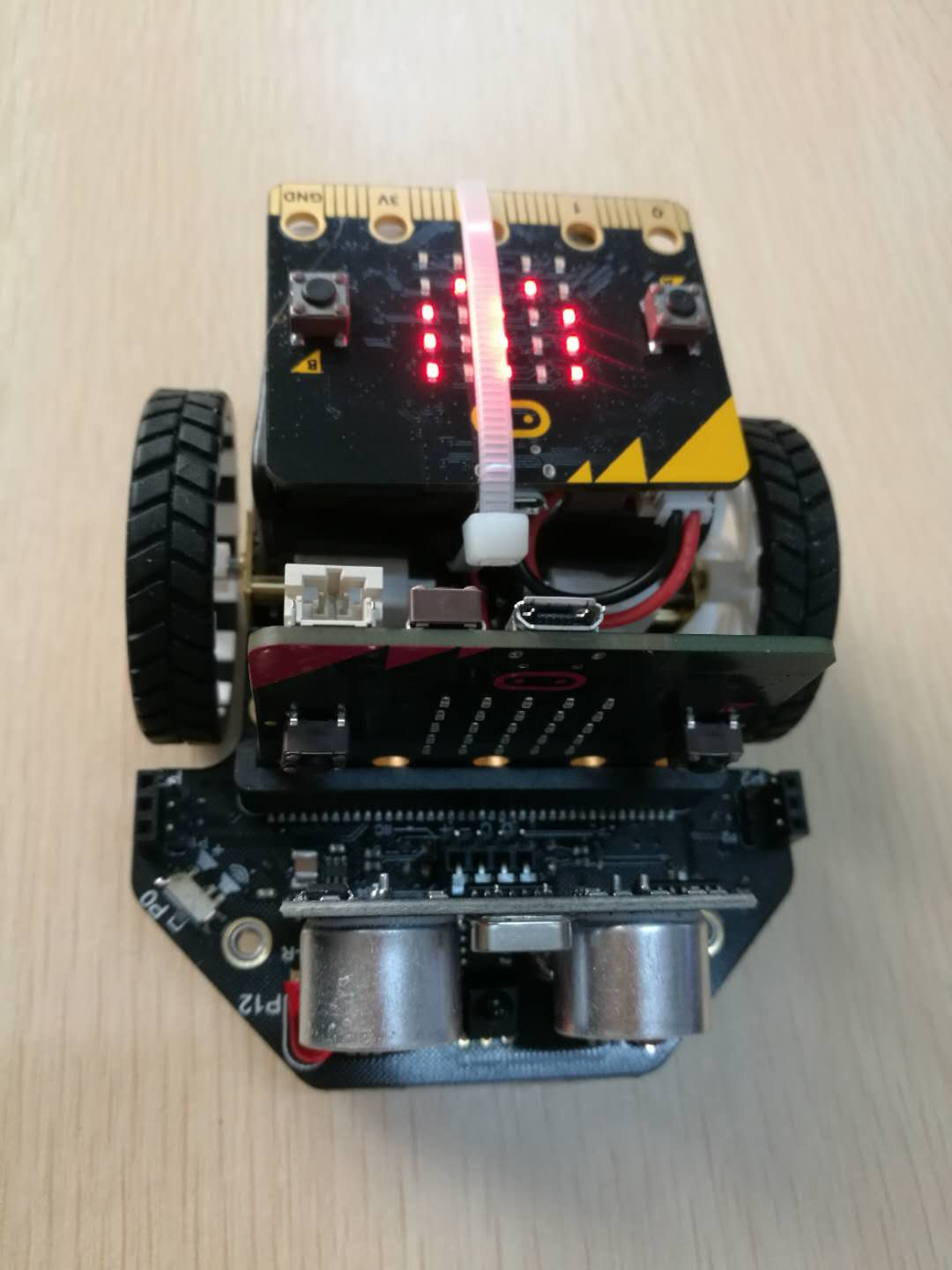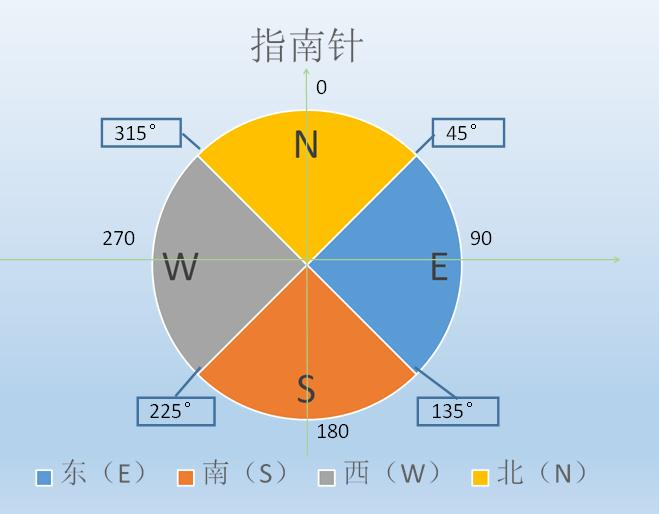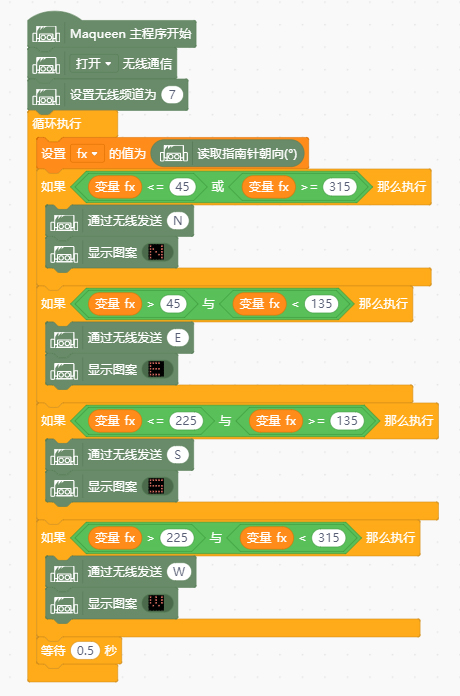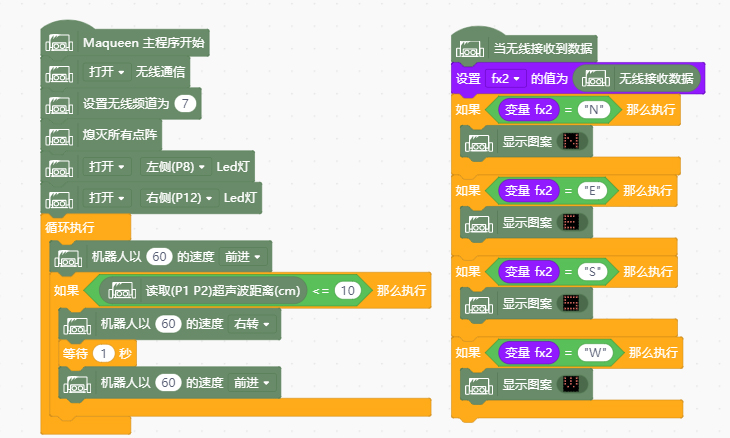[[d.project_title]][[d.material_name]]# 有目标有方向，争当新时代好麦昆

……MICROBIT不是本身就带有电子罗盘吗？1x

1x
MICROBITMICROBIT中可以读取指南针的朝向```	                    					```#include <DFMicrobit_Radio.h>
#include <Microbit_Sensors.h>
#include <Microbit_Matrix.h>

double df_fx;

const uint8_t bitmap_ZmEZ = {B10001,B11001,B10101,B10011,B10001};
const uint8_t bitmap_7XwX = {B11110,B10000,B11110,B10000,B11110};
const uint8_t bitmap_npeC = {B11111,B10000,B11111,B00001,B11111};
const uint8_t bitmap_miMs = {B10101,B10101,B10101,B01010,B00000};

void setup() {
Sensors.calibrate();
}

void loop() {
if (((df_fx <= 45) || (df_fx >= 315))) {
MMatrix.show(bitmap_ZmEZ);
}
if (((df_fx > 45) && (df_fx < 135))) {
MMatrix.show(bitmap_7XwX);
}
if (((df_fx <= 225) && (df_fx >= 135))) {
MMatrix.show(bitmap_npeC);
}
if (((df_fx > 225) && (df_fx < 315))) {
MMatrix.show(bitmap_miMs);
}
delay(500);
}
```
```

（插一句：以前用MIXLY，自从发现MIND+之后，越来越觉得MIND+好用）```	                    					```#include <DFMicrobit_Radio.h>
#include <Microbit_Matrix.h>
#include <Maqueen_Motor.h>
#include <DFRobot_URM10.h>

String df_fx2;

Maqueen_Motor motor;
DFRobot_URM10 urm10(1,2);
const uint8_t bitmap_EHfH = {B10001,B11001,B10101,B10011,B10001};
const uint8_t bitmap_OXOn = {B11110,B10000,B11110,B10000,B11110};
const uint8_t bitmap_Capa = {B11111,B10000,B11111,B00001,B11111};
const uint8_t bitmap_OyOs = {B10101,B10101,B10101,B01010,B00000};

{
df_fx2 = message;
if ((df_fx2 == "N")) {
MMatrix.show(bitmap_EHfH);
}
if ((df_fx2 == "E")) {
MMatrix.show(bitmap_OXOn);
}
if ((df_fx2 == "S")) {
MMatrix.show(bitmap_Capa);
}
if ((df_fx2 == "W")) {
MMatrix.show(bitmap_OyOs);
}
}

void setup() {
MMatrix.clear();
digitalWrite(8,HIGH);
digitalWrite(12,HIGH);
}

void loop() {
motor.motorRun(motor.LEFT,motor.CW,60);
motor.motorRun(motor.RIGHT,motor.CW,60);
if (((urm10.getDistanceCM()) <= 10)) {
motor.motorRun(motor.LEFT,motor.CW,60);
motor.motorRun(motor.RIGHT,motor.CW,0);
delay(1000);
motor.motorRun(motor.LEFT,motor.CW,60);
motor.motorRun(motor.RIGHT,motor.CW,60);
}
}
```
```

……

Makelog作者原创文章，未经授权禁止转载。
1
1[[c.user_name]] [[c.create_time]]
[[c.parent_comment.count]]
|
[[c.comment_content]]
[[r.user.user_name]] [[r.create_time]]
[[r.comment_content]]

5
15
10
13
7
15
8
13
2
5